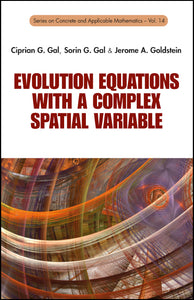# Evolution Equations With A Complex Spatial Variable

Ciprian G Gal

Format: Print Book

ISBN: 9789814590594

• SGD 117.70
Unit price per
Tax included.

This book investigates several classes of partial differential equations of real time variable and complex spatial variables, including the heat, Laplace, wave, telegraph, Burgers, Black-Merton-Scholes, Schrödinger and Korteweg-de Vries equations.The complexification of the spatial variable is done by two different methods. The first method is that of complexifying the spatial variable in the corresponding semigroups of operators. In this case, the solutions are studied within the context of the theory of semigroups of linear operators. It is also interesting to observe that these solutions preserve some geometric properties of the boundary function, like the univalence, starlikeness, convexity and spirallikeness. The second method is that of complexifying the spatial variable directly in the corresponding evolution equation from the real case. More precisely, the real spatial variable is replaced by a complex spatial variable in the corresponding evolution equation and then analytic and non-analytic solutions are sought.For the first time in the book literature, we aim to give a comprehensive study of the most important evolution equations of real time variable and complex spatial variables. In some cases, potential physical interpretations are presented. The generality of the methods used allows the study of evolution equations of spatial variables in general domains of the complex plane.

Format: Hardcover
No of Pages: 204
Imprint: World Scientific
Publication date: 20140319
Series: Series On Concrete And Applicable Mathematics